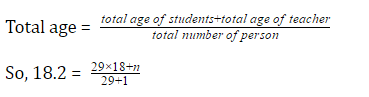# GMAT Quant: Arithmetic – AveragesGMAT is never going to ask you simple questions related to average, rather, they will ask questions based on two or more, different average groups that will be combined together. This is known as weighted average.

There are basically two approaches of solving average questions.

1. Sums & Average
2. Percent & Proportion

Let’s understand these topics in further details:

1. Sums & Averages

Even complex average questions can be solved easily if the Average questions can be seen using the specs of Sums. For example, suppose, there are three sections A, B and C. In which the population of group A is 30%,  population of group B is 60%,  and group C’s population is 10%,  then we can simply pretend that in group C there is only one person, group A is having 3 person and group B has 6 people, and the total population is 10 people.  From this, we can calculate all the sums required.

This method is not the most conventional approach but it can be a life savior at dire need.

1. Percent & Proportion

Usually, you won’t be getting normal statistics, but the values will be in form, of percentage and proportions. This approach is much faster than the sums and average approach. Suppose, we are given percent and their associated averages, then using a simple formula, we can get what we are searching for. Let’s understand this properly:

If, we have got the percentage of three groups and their associated averages, and we have to calculate the total average of all the three groups like here;Then, total average is equal to;

PJ × AJ + PK × AK + PL × AL = Total Average;

Where,

PJ + PK + PL = 1

Let’s solve some questions and understand the concepts of Averages in greater depths:

1. The average age of 29 students is 18. If the age of the teacher is also included, the average age of the class becomes 18.2. Find the age of the teacher?

1. 28
2. 32
3. 22
4. 24

Solution. Average age of 29 students in the class is 18

If the teacher also joins, average becomes 18.2

So, let us assume that the age of teacher is ‘n’.Total age = total age of students+total age of teachertotal number of person

So, 18.2 = 29 × 18 + n29 + 1

n = 24.

This question can be solved using Average pie as well.

Where average × Total number = required value

So, 18 × 29 + n = 546

n = 24.

We’ll be glad to help you in your GMAT preparation journey. You can ask for any assistance related to GMAT and MBA from us by calling us at +918884544444. You can write to us at gmat@byjus.com.